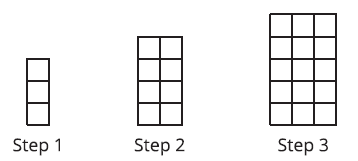# A.6.3.3 Growing StepsSketch the next step in the pattern.

Kiran says that the pattern is growing linearly because as the step number goes up by 1, the number of rows and the number of columns also increase by 1. Do you agree? Explain your reasoning.

To represent the number of squares after steps, Diego and Jada wrote different equations. Diego wrote the equation f(n) = n(n + 2). Jada wrote the equation f(n) = n² + 2n. Are either Diego or Jada correct? Explain your reasoning.

How do you know if the relationship between two quantities represents a quadratic function? What clues would you look for?

Can we say that x(x + 5) and x² + 5x define the same function? How can you show if they do or don’t?